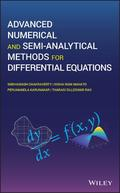John Wiley & Sons Advanced Numerical and Semi-Analytical Methods for Differential EquationsExamines numerical and semi-analytical methods for differential equations that can be used for solvi.. Product #: 978-1-119-42342-3 Regular price: \$95.33 \$95.33 Auf Lager

# Advanced Numerical and Semi-Analytical Methods for Differential Equations1. Auflage Juni 2019
256 Seiten, Hardcover
Wiley & Sons Ltd

ISBN: 978-1-119-42342-3
John Wiley & Sons

Jetzt kaufen

### Preis: 102,00 €

Preis inkl. MwSt, zzgl. Versand

Examines numerical and semi-analytical methods for differential equations that can be used for solving practical ODEs and PDEs

This student-friendly book deals with various approaches for solving differential equations numerically or semi-analytically depending on the type of equations and offers simple example problems to help readers along.

Featuring both traditional and recent methods, Advanced Numerical and Semi Analytical Methods for Differential Equations begins with a review of basic numerical methods. It then looks at Laplace, Fourier, and weighted residual methods for solving differential equations. A new challenging method of Boundary Characteristics Orthogonal Polynomials (BCOPs) is introduced next. The book then discusses Finite Difference Method (FDM), Finite Element Method (FEM), Finite Volume Method (FVM), and Boundary Element Method (BEM). Following that, analytical/semi analytic methods like Akbari Ganji's Method (AGM) and Exp-function are used to solve nonlinear differential equations. Nonlinear differential equations using semi-analytical methods are also addressed, namely Adomian Decomposition Method (ADM), Homotopy Perturbation Method (HPM), Variational Iteration Method (VIM), and Homotopy Analysis Method (HAM). Other topics covered include: emerging areas of research related to the solution of differential equations based on differential quadrature and wavelet approach; combined and hybrid methods for solving differential equations; as well as an overview of fractal differential equations. Further, uncertainty in term of intervals and fuzzy numbers have also been included, along with the interval finite element method. This book:
* Discusses various methods for solving linear and nonlinear ODEs and PDEs
* Covers basic numerical techniques for solving differential equations along with various discretization methods
* Investigates nonlinear differential equations using semi-analytical methods
* Examines differential equations in an uncertain environment
* Includes a new scenario in which uncertainty (in term of intervals and fuzzy numbers) has been included in differential equations
* Contains solved example problems, as well as some unsolved problems for self-validation of the topics covered

Advanced Numerical and Semi Analytical Methods for Differential Equations is an excellent text for graduate as well as post graduate students and researchers studying various methods for solving differential equations, numerically and semi-analytically.

1 Basic Numerical Methods

1.1 Introduction

1.2 Ordinary differential equation

1.3 Euler method

1.4 Improved Euler method

1.5 Runge-Kutta Methods

1.6 Multistep methods

1.7 Higher order ODE

2 Integral Transforms

2.1 Introduction

2.2 Laplace Transform

2.3 Fourier Tranform

3 Weighted Residual Methods

3.1 Introduction

3.2 Collocation method

3.3 Subdomain method

3.4 Least-square method

3.5 Galerkin method

3.6 Comparison of WRMs

4 Boundary Characteristics Orthogonal Polynomials

4.1 Introduction

4.2 Gram Schmidt Orthogonalization Process

4.3 Generation of BCOPs

4.4 Galerkin's Method with BCOPs

4.5 Rayleigh-Ritz Method with BCOP's

5 Finite Difference Method

5.1 Introduction

5.2 Finite Difference Scheme

5.3 Explicit and Implicit Finite Difference Schemes

6 Finite Element Method

6.1 Introduction

6.2 Finite element procedure

6.3 Galerkin finite element method

6.4 Structural analysis using FEM

7 Finite Volume Method

7.1 Introduction

7.2 Discretization Techniques of FVM

7.3 General Form of Finite Volume Method

7.4 One Dimensional Convection-diffusion problem

8 Boundary Element Method

8.1 Introduction

8.2 Boundary Representation and Background Theory of BEM

8.3 Derivation of the Boundary Element Method

9 Akbari Ganji's Method

9.1 Introduction

9.2 Nonlinear ordinary differential equations

9.3 Numerical examples

10 Exp-function Method

10.1 Introduction

10.2 Basics of Exp-fucntion method

10.3 Numerical examples

11.1 Introduction

11.2 ADM for Ordinary Differential Equations (ODEs)

11.3 Solving system of ODEs by ADM

11.4 ADM for solving Partial Differential Equations (PDEs)

11.5 ADM for system of PDEs

12 Homotopy Perturbation Method

12.1 Introduction

12.2 Basic idea of HPM

12.3 Numerical examples

13 Variational Iteration Method

13.1 Introduction

13.2 VIM procedure

13.3 Numerical examples

14 Homotopy Analysis Method

14.1 Introduction

14.2 HAM procedure

14.3 Numerical examples

15.1 Introduction

15.2 DQM procedure

15.3 Numerical examples

16 Wavelet Method

16.1 Introduction

16.2 Haar wavelet

16.3 Wavelet-collocation method

17 Hybrid Methods

17.1 Introduction

17.2 Homotopy Perturbation Transform Method

18 Preliminaries of Fractal Differential Equations

18.1 Introduction to fractal

18.2 Fractal differential equations

19 Differential Equations with Interval Uncertainty

19.1 Introduction

19.2 Interval Differential Equations (IDEs)

19.3 Generalized Hukuhara Differentiability of IDEs

19.4 Analytical Methods for IDEs

20 Differential Equations with Fuzzy Uncertainty

20.1 Solving Fuzzy Linear System of Differential Equations

21 Interval Finite Element Method

21.1 Introduction

21.2 Interval Galerkin FEM

21.3 Structural analysis using IFEM
SNEHASHISH CHAKRAVERTY, PHD, is Professor in the Department of Mathematics at National Institute of Technology, Rourkela, Odisha, India. He is also the author of Fuzzy Arbitrary Order System: Fuzzy Fractional Differential Equations and Applications and 12 other books.

NISHA RANI MAHATO is a Senior Research Fellow in the Department of Mathematics at the National Institute of Technology, Rourkela, Odisha, India where she is pursuing her PhD.

PERUMANDLA KARUNAKAR is a Senior Research Fellow in the Department of Mathematics at the National Institute of Technology, Rourkela, Odisha, India where he is pursuing his PhD.

THARASI DILLESWAR RAO, is a Senior Research Fellow in the Department of Mathematics at the National Institute of Technology, Rourkela, Odisha, India where he is pursuing his PhD.